# Tag Archives: simulation

A technique succeeds in mathematical physics, not by a clever trick, or a happy accident, but because it expresses some aspect of physical truth (O. G. Sutton)

Imagine three unbalanced coins:

• Coin 1: Probability of head=0.495 and probability of tail=0.505
• Coin 2: Probability of head=0.745 and probability of tail=0.255
• Coin 3: Probability of head=0.095 and probability of tail=0.905

Now let’s define two games using these coins:

• Game A: You toss coin 1 and if it comes up head you receive 1€ but if not, you lose 1€
• Game B: If your present capital is a multiple of 3, you toss coin 2. If not, you toss coin 3. In both cases, you receive 1€ if coin comes up head and lose 1€ if not.

Played separately, both games are quite unfavorable. Now let’s define Game A+B in which you toss a balanced coin and if it comes up head, you play Game A and play Game B otherwise. In other words, in Game A+B you decide between playing Game A or Game B randomly.

Starting with 0€, it is easy to simulate the three games along 500 plays. This is an example of one of these simulations: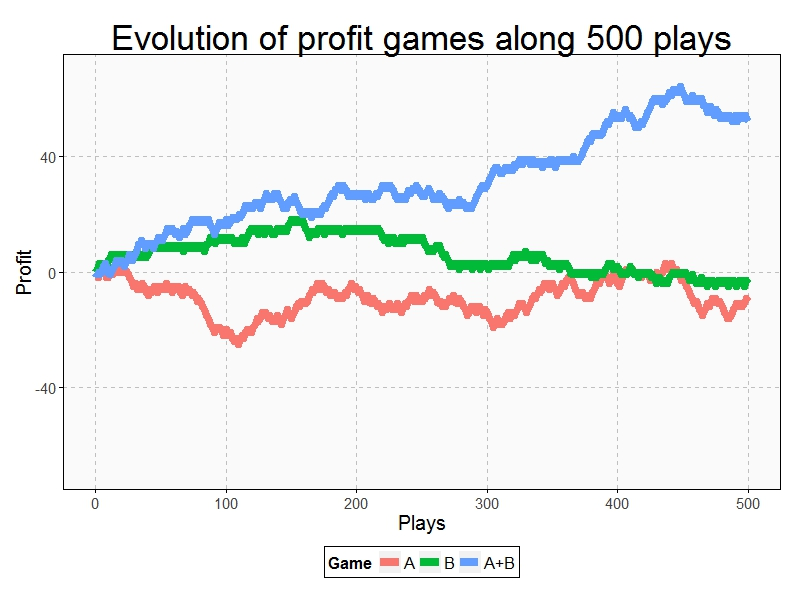Resulting profit of Game A+B after 500 plays  is +52€ and is -9€ and -3€ for Games A and B respectively. Let’s do some more simulations (I removed legends and titles but colors of games are the same):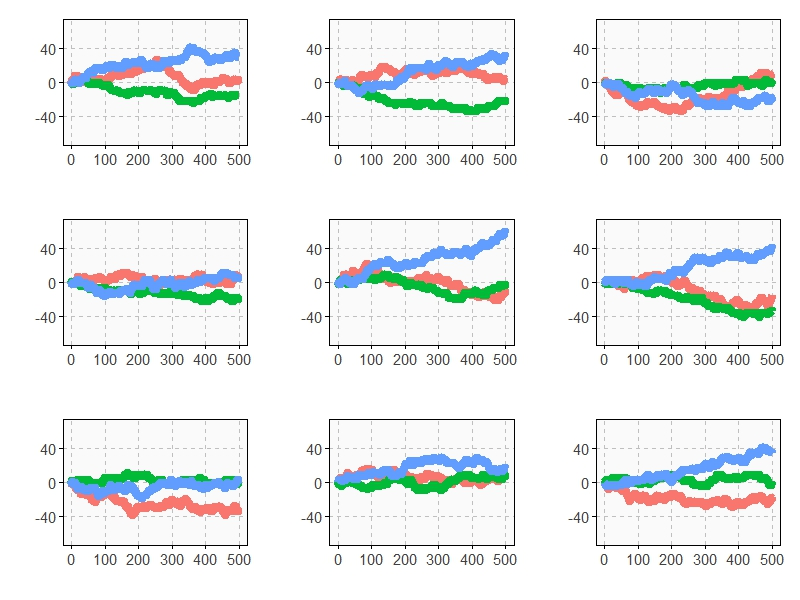As you can see, Game A+B is the most profitable in almost all the previous simulations. Coincidence? Not at all. This is a consequence of the stunning Parrondo’s Paradox which states that two losing games can combine into a winning one.

If you still don’t believe in this brain-crashing paradox, following you can see the empirical distributions of final profits of three games after 1.000 plays: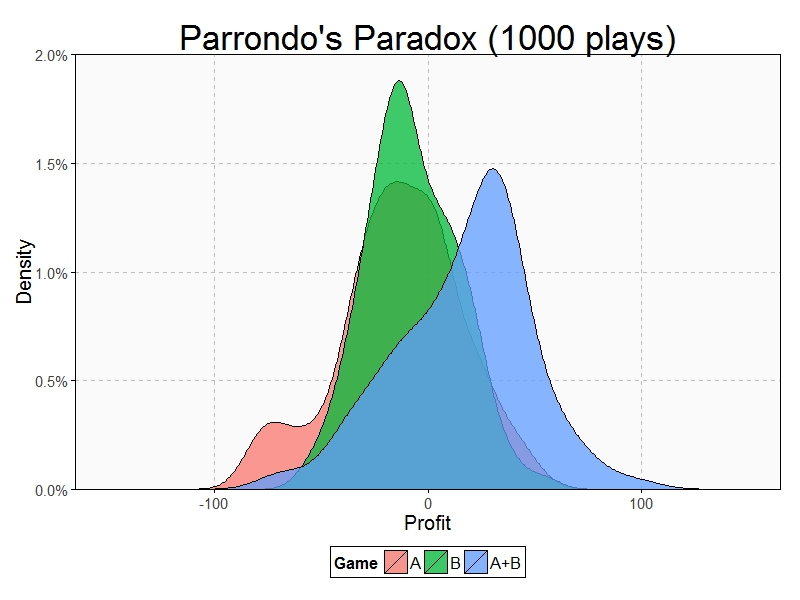After 1000 plays, mean profit of Game A is -13€, is -7€ for Game B and 17€ for Game A+B.

This paradox was discovered in the last nineties by the Spanish physicist Juan Parrondo and can help to explain, among other things, why investing in losing shares can result in obtaining big profits. Amazing:

```require(ggplot2)
require(scales)
library(gridExtra)
opts=theme(
legend.position = "bottom",
legend.background = element_rect(colour = "black"),
panel.background = element_rect(fill="gray98"),
panel.border = element_rect(colour="black", fill=NA),
axis.line = element_line(size = 0.5, colour = "black"),
axis.ticks = element_line(colour="black"),
panel.grid.major = element_line(colour="gray75", linetype = 2),
panel.grid.minor = element_blank(),
axis.text.y = element_text(colour="gray25", size=15),
axis.text.x = element_text(colour="gray25", size=15),
text = element_text(size=20),
plot.title = element_text(size = 35))
PlayGameA = function(profit, x, c) {if (runif(1) < c-x) profit+1 else profit-1}
PlayGameB = function(profit, x1, c1, x2, c2) {if (profit%%3>0) PlayGameA(profit, x=x1, c=c1) else PlayGameA(profit, x=x2, c=c2)}
####################################################################
#EVOLUTION
####################################################################
noplays=500
alpha=0.005
profit0=0
results=data.frame(Play=0, ProfitA=profit0, ProfitB=profit0, ProfitAB=profit0)
for (i in 1:noplays) {results=rbind(results, c(i,
PlayGameA(profit=results[results\$Play==(i-1),2], x =alpha, c =0.5),
PlayGameB(profit=results[results\$Play==(i-1),3], x1=alpha, c1=0.75, x2=alpha, c2=0.1),
if (runif(1)<0.5) PlayGameA(profit=results[results\$Play==(i-1),4], x =alpha, c =0.5) else PlayGameB(profit=results[results\$Play==(i-1),4], x1=alpha, c1=0.75, x2=alpha, c2=0.1)
))}
results=rbind(data.frame(Play=results\$Play, Game="A",   Profit=results\$ProfitA),
data.frame(Play=results\$Play, Game="B",   Profit=results\$ProfitB),
data.frame(Play=results\$Play, Game="A+B", Profit=results\$ProfitAB))
ggplot(results, aes(Profit, x=Play, y=Profit, color = Game)) +
scale_x_continuous(limits=c(0,noplays), "Plays")+
scale_y_continuous(limits=c(-75,75), expand = c(0, 0), "Profit")+
labs(title="Evolution of profit games along 500 plays")+
geom_line(size=3)+opts
####################################################################
#DISTRIBUTION
####################################################################
noplays=1000
alpha=0.005
profit0=0
results2=data.frame(Play=numeric(0), ProfitA=numeric(0), ProfitB=numeric(0), ProfitAB=numeric(0))
for (j in 1:100) {results=data.frame(Play=0, ProfitA=profit0, ProfitB=profit0, ProfitAB=profit0)
for (i in 1:noplays) {results=rbind(results, c(i,
PlayGameA(profit=results[results\$Play==(i-1),2], x =alpha, c =0.5),
PlayGameB(profit=results[results\$Play==(i-1),3], x1=alpha, c1=0.75, x2=alpha, c2=0.1),
if (runif(1)<0.5) PlayGameA(profit=results[results\$Play==(i-1),4], x =alpha, c =0.5)
else PlayGameB(profit=results[results\$Play==(i-1),4], x1=alpha, c1=0.75, x2=alpha, c2=0.1)))}
results2=rbind(results2, results[results\$Play==noplays, ])}
results2=rbind(data.frame(Game="A", Profit=results2\$ProfitA),
data.frame(Game="B", Profit=results2\$ProfitB),
data.frame(Game="A+B", Profit=results2\$ProfitAB))
ggplot(results2, aes(Profit, fill = Game)) +
scale_x_continuous(limits=c(-150,150), "Profit")+
scale_y_continuous(limits=c(0,0.02), expand = c(0, 0), "Density", labels = percent)+
geom_density(alpha=.75)+opts
```

# Batman’s Choice

A hero can be anyone, even a man doing something as simple and reassuring as putting a coat on a young boy’s shoulders to let him know the world hadn’t ended (Batman in The Dark Knight Rises)

Joker has captured Batman and keeps him into a dark and cold dungeon of Gotham City. Showing his sadistic character, Joker proposes the following game to Batman:

This is a six shooter revolver with two bullets in the cylinder. Bullets are inside two consecutive chambers. I will spin the cylinder and will fire the gun aiming to my head. If I survive you will have to do the same but you decide if you want to spin the cylinder before firing or not. If you still keep you head over your shoulders after firing, you will be free.

Joker fires and nothing happens. He survives and passes the revolver to Batman. What should Batman do? Spinning or not? What would you do?

From my point of view, answer is quite anti-intutive because the best option is not spinning the cylinder again. Spinning case is clear: probability of losing the head is 2/6=33% but what about not spinning? Doing the next shoot directly eliminates two possibilities: the previous shot of Joker and the second bullet according to direction of cylinder rotation (remember two bullets are consecutive and Joker is unfortunately  still alive). It means there is only 1 chance to dead between 4, so probability of losing the head in this scenario is 1/4=25% which is significantly lower than the first one.

Here you can find the resulting graph of simulating the game up to 500 times:Will it be the end of Batman? Not sure.

This is the code of this experiment:

```library(ggplot2)
library(extrafont)
niter=500
results=data.frame()
for (i in 1:niter)
{
bullet1=sample(1:6,1)
Joker=sample((1:6)[-c(bullet1, bullet1%%6+1)],1)
#Option 1: Shooting
Batman1=Joker%%6+1
#Option 2: Rolling and Shooting
Batman2=sample(1:6,1)
}
theme_xkcd=theme(
panel.background = element_rect(fill="darkolivegreen1"),
panel.border = element_rect(colour="black", fill=NA),
axis.line = element_line(size = 0.5, colour = "black"),
axis.ticks = element_line(colour="black"),
panel.grid = element_line(colour="white", linetype = 2),
axis.text.y = element_text(colour="black"),
axis.text.x = element_text(colour="black"),
text = element_text(size=18, family="Humor Sans"),
plot.title = element_text(size = 50)
)
p=ggplot(data=results, aes(x=iter, y=csum1))+
geom_abline(intercept = 1/4, slope = 0, size = 0.4, linetype=2, colour = "black", alpha=0.8)+
geom_abline(intercept = 1/3, slope = 0, size = 0.4, linetype=2, colour = "black", alpha=0.8)+
geom_line(aes(y=csum2), colour="green4", size=1.5, fill=NA)+
geom_line(colour="green4", size=1.5, fill=NA)+
coord_cartesian(ylim=c(.0, 1), xlim=c(1, niter))+
scale_y_continuous(breaks = c(0,round(1/4, digits = 2),round(1/3, digits = 2),1))+
geom_text(data=results[niter*.75, ], family="Humor Sans", colour="green4", y=0.38, label="Rotating Cylinder and Shooting ...", size=4, adjust=1)+
geom_text(data=results[niter*.75, ], family="Humor Sans", colour="green4", y=0.20, label="Shooting without Rotating Cylinder ...", size=4, adjust=1)+
labs(x="Number Of Trials", y="Prob. of Losing The Head", title="Batman's Choice")+
theme_xkcd
ggsave("batmans_choice.jpg", plot=p, width=8, height=5)
```

# Do Not Play With Mr. Penney

Facts do not speak (Henry Poincare)

Mr. Penney is my best friend. He is maths teacher and loves playing. Yesterday we were in his office at the university when he suggested me a game:

When you toss a coin three times, you can obtain eight different sequences of tails and heads: TTT, TTH, THT, HTT, THH, HTH, HHT and HHH. Using a fair coin, all sequences have the same chances to appear. Choose one sequence and I will then choose another one. I will toss a coin until either your or my sequence appears as a consecutive subsequence of the coin toss outcomes. The player whose sequence appears first wins. I will repeat this procedure 100 times. The one with more games won is the winner of the game.  Don’t worry: I will not toss the coin manually. I will simulate using my computer. What’s your bet?

Ok, my bet is THT, I said. After some seconds, Mr. Penney said: My bet is TTH.

This was the result of the first round: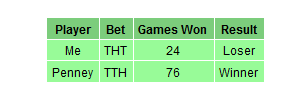Another chance? told me Mr. Penney. Of course! Now my bet is TTH! I said. In fact, I was thinking Take that! Now I chose your previous bet. Do you think I am foolish?. After some seconds, Mr. Penney said: My bet now is HTT.

This was the result of the second round:Another chance? told me Mr. Penney. At this point, I was very suspicious but I wanted the last chance so I told him Of course! Now my bet is HTT! I wanted to try my strategy one more time. After some seconds, Mr. Penney said: My bet now is HHT.

This was the result of the third round:Ok, I give it up! What’s the trick? I said. And Mr. Penney explained it to me. You can find the explanation here. This is the last time I play with you! I told him once he finished the explanation.

Here you have the code. Feel free to play:

```library(gridExtra)
library(gridExtra)
Me     <- "TTH"
Penney <- "HTT"
results <- data.frame(play= numeric(0), Penney = integer(0), Me = character(0))
for (i in 1:100) {
play <- c()
repeat {play <- do.call(paste, c(play, as.list(sample(c("H","T"), 1)), sep=""))
if (grepl(Penney, play)|grepl(Me, play)) {
results <- rbind(results, data.frame(play= i, Penney = as.numeric(grepl(Penney, play)), Me = as.numeric(grepl(Me, play))))
break}}}
grid.newpage()
table <- rbind(
c("Me", Me, sum(results\$Me), if(sum(results\$Penney) > sum(results\$Me)) "Loser" else "Winner"),
c("Penney", Penney, sum(results\$Penney), if(sum(results\$Penney) > sum(results\$Me)) "Winner" else "Loser"))
grid.table(table,
cols = c("Player", "Bet", "Games Won", "Result"),
gpar.colfill = gpar(fill="palegreen3",col="White"),
gpar.corefill =  gpar(fill="palegreen",col="White"),
gpar.rowfill = gpar(fill=NA, col=NA))
```

# Random Love

Anyone who considers arithmetical methods of producing random digits is, of course, in a state of sin (John von Newman)

Ms. Positive and Mr. Negative live in a one-dimensional world and are falling in love. But beginnings are not always easy. They have a big problem: none of them like the other’s neighborhood. Ms. Positive only wants to walk around Positive Integer Numbers Neighborhood and Mr. Negative around Negative Integers Numbers one. This is a prickly problem they need to deal with as soon as possible. But they have a good idea. They will start their walks from Zero, an impartial place between both neighborhoods and will let fate to guide their feet. They will toss a coin to decide every step: if result is head, they will advance 1 step toward positive numbers neighborhood; if tail, they will advance 1 step toward negative numbers one. For example, if the first 5 tosses are face, face, tail, tail and tail, the their first 5 steps will be +1, +2, +1, 0 and -1. It seems to be a fair agreement for both. Maybe is not the most pleasant way to take a walk but It is well known that lovers use to do silly things constantly, especially at the beginnings. They always walk for two hours, so they toss the coin 7.200 times every walk (these lovers are absolutely crazy as you can see). This was their first walk:After this first walk, Mr Negative was really upset. Ms. Positive, watching his face fell, ask him: What’s the matter, honey? and Mr. Negative replied: What’s the matter? What’s the matter? The matter is that we spent almost all the time walking around your horrible neighborhood! What comes next is too hard to be reproduced here. Anyway, they agreed to give a chance to the method they designed. How can one imagine that a coin can produce such a strange walk! There must be an error! After 90 walks, the situation of our lovers was extremely delicate. A 57% of the walks were absolutely awful for one of them since more than 80% of the steps were around the same neighborhood. Another 32% were a bit uncomfortable for one of them since between 60% and 80% of the steps were around the same neighborhood. Only 11% of the walks were gratifying. How is it possible?, said Mr. Negative. How is it possible?, said Ms. Positive.But here comes Ms. Positive, who always looks on the brigth side of life: Don’t worry, darling. In fact, we don’t have to be sad. We get angry the same amount of times! For me is enough. What about you?, said her. For me is perfect as well!, said Mr. Negative. In that moment, they realise they were made for each other and started another random walk with a big smile on their faces.This is the code:

```library(ggplot2)
steps   <- 2*60*60 #Number of steps
results <- data.frame()
walks<-90 #Number of walks
for (i in 1:walks)
{
state <- cumsum(sample(c(-1,1), steps, replace = TRUE))
results <- rbind(results, c(sum(state<0), sum(state>0), sum(state==0),
if (sum(state<0) >= sum(state>0)) 1 else 0))
}
colnames(results) <- c("neg.steps", "pos.steps", "zero.steps", "ind.neg")
results\$max.steps <- apply(results, 1, max)/apply(results, 1, sum)
#Plot of one of these walks
mfar=max(abs(max(state)),abs(min(state)))
plot1 <- qplot(seq_along(state),
state,
geom="path")+
xlab("Step") +
ylab("Location") +
labs(title = "The First Walk Of Ms. Positive And Mr. Negative")+
theme(plot.title = element_text(size = 35))+
theme(axis.title.y = element_text(size = 20))+
theme(axis.title.x = element_text(size = 20))+
scale_x_continuous(limits=c(0, length(state)),breaks=c(1,steps/4,steps/2,3*steps/4,steps))+
scale_y_continuous(limits=c(-mfar, mfar), breaks=c(-mfar,-mfar/2, 0, mfar/2,mfar))+
geom_hline(yintercept=0)
ggsave(plot1, file="plot1.png", width = 12, height = 10)
#Summary of all walks
hist1 <- ggplot(results, aes(x = max.steps))+
geom_histogram(colour = "white",breaks=seq(.4,1,by=.2),fill=c("blue", "orange", "red"))+
theme_bw()+
labs(title = paste("What Happened After ", toString(walks), " Walks?",sep = ""))+
scale_y_continuous(breaks=seq(0,(nrow(results[results\$max.steps>.8,])+10),by=10))+
theme(plot.title = element_text(size = 40))+
xlab("Maximum Steps In The Same Location (%)") +
ylab("Number of Walks")
ggsave(hist1, file="hist1.png", width = 10, height = 8)
#Data for waterfall chart
waterfall <- as.data.frame(cbind(
c("Total Walks", "Satisfactory Walks", "Uncomfortable Walks", "Awful Walks for Mr. +", "Awful Walks for Ms. -"),
c("a", "b", "c", "d", "d"),
c(0,
nrow(results),
nrow(results)-nrow(results[results\$max.steps<.6,]),
nrow(results)-nrow(results[results\$max.steps<.6,])-nrow(results[results\$max.steps>=.6 & results\$max.steps<.8,]),
nrow(results)-nrow(results[results\$max.steps<.6,])-nrow(results[results\$max.steps>=.6 & results\$max.steps<.8,])-nrow(results[results\$max.steps>=.8 & results\$ind.neg==1,])
),
c(nrow(results),
nrow(results)-nrow(results[results\$max.steps<.6,]),
nrow(results)-nrow(results[results\$max.steps<.6,])-nrow(results[results\$max.steps>=.6 & results\$max.steps<.8,]),
nrow(results)-nrow(results[results\$max.steps<.6,])-nrow(results[results\$max.steps>=.6 & results\$max.steps<.8,])-nrow(results[results\$max.steps>=.8 & results\$ind.neg==1,]),
0
),
c(nrow(results),
nrow(results[results\$max.steps<.6,]),
nrow(results[results\$max.steps>=.6 & results\$max.steps<.8,]),
nrow(results[results\$max.steps>=.8 & results\$ind.neg==1,]),
nrow(results[results\$max.steps>=.8 & results\$ind.neg==0,]))
))
colnames(waterfall) <-c("desc", "type", "start", "end", "amount")
waterfall\$id <- seq_along(waterfall\$amount)
waterfall\$desc <- factor(waterfall\$desc, levels = waterfall\$desc)
#Waterfall chart
water1 <- ggplot(waterfall, aes(desc, fill = type)) +
geom_rect(aes(x = desc, xmin = id-0.45, xmax = id+0.45, ymin = end, ymax = start))+
xlab("Kind of Walk") +
ylab("Number of Walks") +
labs(title = "The Ultimate Proof (After 90 Walks)")+
theme(plot.title = element_text(size = 35))+
theme(axis.title.y = element_text(size = 20))+
theme(axis.title.x = element_text(size = 20))+
theme(legend.position = "none")
```

# Dora’s Choice

Arithmetic is being able to count up to twenty without taking off your shoes (Mickey Mouse)On her last mission, Dora The Explorer sails down the Amazon river to save her friend Isa The Iguana from Swiper The Fox claws. After some hours of navigation, Dora sees how the river divides into 3 branches and has to choose which one to follow. Before leaving, her friend Map told her that just one of these branches is safe. Two others end in terrible waterfalls, both impossible to escape alive. Although Dora does not know which one is the good one, she decides to take the branch number 1. Suddenly, her friend Boots The Monkey yells from the top of a palm tree:

Dora, do not take branch number 3! I can see from here that it ends in a horrible waterfall!

After listening to Boots, Dora changes her mind and decides to take branch number 2. Why Dora switches? Because she knows that this change has significantly increased her probability of ending the mission alive.

There are several ways to convince yourself of this. One is to simulate the situation that has faced Dora and compare results of switching and not switching . Switching, Dora saves her life 2 of each 3 simulations while if she does not, Dora only saves 1 of each 3 simulations. Changing her mind, Dora doubles her chances of survival!

Carefully considering what happens, you can see that switching Dora saves herself when her first choice is erroneus, which occurs with probability 2/3. On the other hand, if Dora remains faithful to her first choice, obviously only saves herself with probability 1/3.

This is an example on my own of the famous Monty Hall Problem. You can see a nice explanation of it in a chapter of Numb3rs or in the film 21 Black Jack. Not long ago I exposed the problem in a family meeting. Only my mum said she would switch (we were 6 people in the meeting). It is fun to share this experiment and ask what people would do. Do it with your friends and family. First time I knew the problem I thought there were no difference between switching and not since I gave both possibilities 1/2 of probability. If I had been Dora, pretty sure I would tumbled over a terrible waterfall. What about yo?

Note: this is an update of the post, which was not a correct formulation of Monty Hall Problem. Thanks to David Robinson and Scott Kostyshak for showing me my error. A correct formulation of the problem may be this:

On her last mission, Dora The Explorer sails down the Amazon river to meet her cousin Diego. After some hours of navigation, Dora sees how the river divides into 3 branches and has to choose which one to follow. Before leaving, her friend Map told her that just one of these branches is safe. Two others end in terrible waterfalls, both impossible to escape alive. Although Dora does not know which one is the good one, she decides to take the branch number 1. After putting the bow towards branch number one, Dora sees Swiper The Fox smiling from the shore, in a high place where obviously can see the end of all three branches. Dora yells him:

– Help me Swiper! Which one should I take?

Swiper replies:

– I am the villain of this story so I will give you only an advice: do not take branch number 3. It ends into a terrible waterfall.

Dora, who has a sixth sense to notice when Swiper is lying, knows he is telling the truth and immediately changes her mind and decides to take branch number 2. Why Dora switches? Because she knows that this change has significantly increased her probability of ending the mission alive.Here you have the code:

```library(ggplot2)
library(extrafont)
nchoices <- 3
nsims <- 500
choices <- seq(from=1, to=nchoices, by=1)
good.choice <- sample(choices, nsims, replace=TRUE)
choice1 <- sample(choices, nsims, replace=TRUE)
dfsims <- as.data.frame(cbind(good.choice, choice1))
dfsims\$advice <- apply(dfsims, 1, function(x) choices[!choices %in% as.vector(x)][sample(1:length(choices[!choices %in% as.vector(x)]), 1)])
dfsims\$choice2 <- apply(dfsims, 1, function(x) choices[!choices %in% as.vector(c(x, x))][sample(1:length(choices[!choices %in% as.vector(c(x, x))]), 1)])
dfsims\$win1 <- apply(dfsims, 1, function(x) (x==x)*1)
dfsims\$win2 <- apply(dfsims, 1, function(x) (x==x)*1)
dfsims\$csumwin1 <- cumsum(dfsims\$win1)/as.numeric(rownames(dfsims))
dfsims\$csumwin2 <- cumsum(dfsims\$win2)/as.numeric(rownames(dfsims))
dfsims\$nsims <- as.numeric(rownames(dfsims))
dfsims\$xaxis <- 0
### XKCD theme
theme_xkcd <- theme(
panel.background = element_rect(fill="darkolivegreen1"),
panel.border = element_rect(colour="black", fill=NA),
axis.line = element_line(size = 0.5, colour = "black"),
axis.ticks = element_line(colour="black"),
panel.grid = element_line(colour="white", linetype = 2),
axis.text.y = element_text(colour="black"),
axis.text.x = element_text(colour="black"),
text = element_text(size=18, family="Humor Sans"),
plot.title = element_text(size = 50)
)
### Plot the chart
p <- ggplot(data=dfsims, aes(x=nsims, y=csumwin1))+
geom_line(aes(y=csumwin2), colour="green4", size=1.5, fill=NA)+
geom_line(colour="green4", size=1.5, fill=NA)+
geom_text(data=dfsims[400, ], family="Humor Sans", aes(x=nsims), colour="green4", y=0.7, label="if Dora switches ...", size=5.5, adjust=1)+
geom_text(data=dfsims[400, ], family="Humor Sans", aes(x=nsims), colour="green4", y=0.3, label="if Dora does not switch ...", size=5.5, adjust=1)+
coord_cartesian(ylim=c(0, 1), xlim=c(1, nsims))+
scale_y_continuous(breaks = c(0,round(1/3, digits = 2),round(2/3, digits = 2),1), minor_breaks = c(round(1/3, digits = 2),round(2/3, digits = 2)))+
scale_x_continuous(minor_breaks = seq(100, 400, 100))+
labs(x="Number Of Simulations", y="Rate Of Survival", title="Dora's Choice")+
theme_xkcd
ggsave("doras_choice.jpg", plot=p, width=8, height=5)
```

# Shoot The Heart With Monte Carlo

The heart has its reasons which reason knows not (Blaise Pascal)

You only need two functions to draw a heart mathematically. The upper part is generated by `(1-(|x|-1)2)1/2` and the lower one by `acos(1-|x|)-PI`. Here is how this heart is: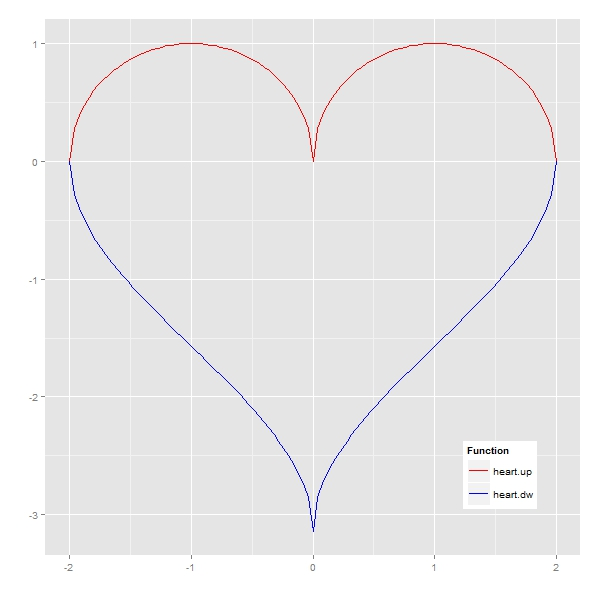Whats the area of this heart? It’s easy: integrating `heart.up(x)-heart.dw(x)` between -2 and 2 and you will obtain that heart measures 9.424778, but there is a simple and nice way to approximate to this value: shoot the heart.

The idea is very simple. Heart is delimited by a square with vertex in `(-2, heart.dw(0))`, `(-2, 1)`, `(2, heart.dw(0))` and `(2, 1)`. Generating a set of points uniformly distributed inside the square and counting how many of them fall into the heart in relation to the area of the square gives a very good approximation of the exact area of the heart. This is a plot representing a simulation of 2.000 shots (hits in red, fails in blue):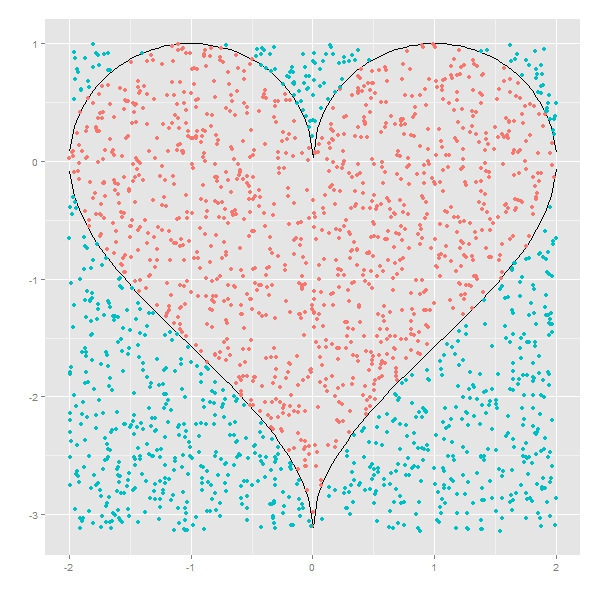Given a simulation of n points, the estimated area of the heart is the area of the square by percentage of points that falls inside the heart. And of course, precision increases with the number of shots you make, as you can see in the following plot, where exact area is represented by the red horizontal line:Here you have the code:

```library("ggplot2")
heart.up <- function(x) {sqrt(1-(abs(x)-1)^2)} #Upper part of the heart
heart.dw <- function(x) {acos(1-abs(x))-pi}    #Lower part of the heart
#Plot of the heart
ggplot(data.frame(x=c(-2,2)), aes(x)) +
stat_function(fun=heart.up, geom="line", aes(colour="heart.up")) +
stat_function(fun=heart.dw, geom="line", aes(colour="heart.dw")) +
scale_colour_manual("Function", values=c("blue","red"), breaks=c("heart.up","heart.dw"))+
labs(x = "", y = "")+
theme(legend.position = c(.85, .15))
sims <- 2000 #Number of simulations
rlts <- data.frame()
for (i in 1:sims)
{
msm <- cbind(as.matrix(runif(i, min=-2, max=2)), as.matrix(runif(i, min=heart.dw(0), max=1)))
nin <- 0
for (j in 1:nrow(msm)) {if (msm[j,2]<=heart.up(msm[j,1]) & msm[j,2]>=heart.dw(msm[j,1])) nin=nin+1}
rlts <- rbind(c(i, 4*(1-heart.dw(0))*nin/i), rlts)
}
colnames(rlts) <- c("no.simulations","heart.area")
exact.area <- integrate(function(x) {heart.up(x)-heart.dw(x)},-2,2)\$value
mean.area <- mean(rlts\$heart.area) #Mean of All Estimated Areas
ggplot(data = rlts, aes(x = no.simulations, y = heart.area))+
geom_point(size = 0.5, colour = "black", alpha=0.4)+
geom_abline(intercept = exact.area, slope = 0, size = 1, linetype=1, colour = "red", aes(color="My Line"), alpha=0.8, show_guide = TRUE)+
labs(list(x = "Number of Shots", y = "Estimated Area"))+
ggtitle("Shot The Heart With Monte Carlo") +
theme(plot.title = element_text(size=20, face="bold"))+
scale_x_continuous(limits = c(0, sims), expand = c(0, 0))+
expand_limits(x = 0, y = 0)+
scale_y_continuous(limits = c(0, 2*exact.area), expand = c(0, 0), breaks=c(0, exact.area/4, exact.area/2, 3*exact.area/4, exact.area, 5*exact.area/4, 3*exact.area/2, 7*exact.area/4, 2*exact.area))+
geom_text(x = 1000, y = exact.area/2, label=paste("Exact Area =", sprintf("%7.6f", exact.area)), vjust=-1, colour="red", size=5)+
geom_text(x = 1000, y = exact.area/2, label=paste("Mean of All Estimated Areas=", sprintf("%7.6f", mean.area)), vjust=+1, colour="red", size=5)
```